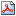# How and When Should One Control for Endogenity Biases? Part I: The Impact of a Possibly Endogenous Explanatory Variable on a Continuous Outcomewp-03-69.pdf — PDF document, 1,016 kB (1,041,327 bytes)

Author(s): Angeles G, Guilkey D K, Mroz T A

Year: 2003

Abstract:
The interpretation of coefficients estimates from ordinary least square regressions and other statistical models depends crucially on whether any explanatory variable in the statistical model is correlated with the ôerror termö influencing the outcome of interest. If there is a relationship between any explanatory variable and the unmeasured determinants of an outcome, then one usually cannot interpret any of the estimated coefficients as the impact of the corresponding covariate on the outcome of interest. In the medical and public health literature, this is often called the problem of confounding effects. In economics and sociology, one typically calls this the problem of endogenous regressors. Regardless of the label chosen for this relationship, the presence of a correlation between the measured and unmeasured determinants of an outcome results in biased estimators of the impacts of all covariates. In this paper we explore the severity of the possible biases that can arise when such correlations are present, and we examine the performance of some simple estimators that have been developed to reduce the bias. We start out by examining ordinary least square models with continuous outcomes and continuous regressors because most of the intuition about the problems and the solutions can be developed simply in that context. We then examine endogeneity problems and solutions for three other sets of models that researchers often encounter in practice: a continuous outcome influenced by an endogenous binary regressor; a binary (discrete) outcome determined by an endogenous continuous regressor; and a binary outcome being influenced by an endogenous binary regressor. In nearly all instances we focus on the estimation of the impact of the possibly endogenous regressor on the outcome of interest, but it is important to recognize that estimators for all effects in a model, not just those for the endogenous variables, usually are biased when any explanatory variable is endogenous. We also examine the performance of estimators in situations where the researcher cares about more than just the bias of the estimator.

Filed under: Public Health